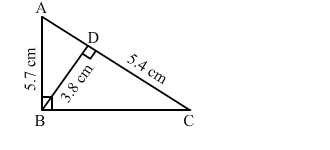# In the given figure, ∠ABC = 90° and BD ⊥ AC.

Question:

In the given figure, ∠ABC = 90° and BD ⊥ AC. If AB = 5.7 cm, BD = 3.8 cm and CD = 5.4 cm, find BC.Solution:

It is given that ABC is a right angled triangle and BD is the altitude drawn from the right angle to the hypotenuse.

In $\triangle B D C$ and $\triangle A B C$, we have :

$\angle A B C=\angle B D C=90^{\circ}$ (given)

$\angle C=\angle C$ (common)

By AA similarity theorem, we get:

$\triangle B D C \sim \triangle A B C$

$\frac{A B}{B D}=\frac{B C}{D C}$

$\Rightarrow \frac{5.7}{3.8}=\frac{B C}{5.4}$

$\Rightarrow B C=\frac{5.7}{3.8} \times 5.4$

$=8.1$

Hence, BC = 8.1 cm